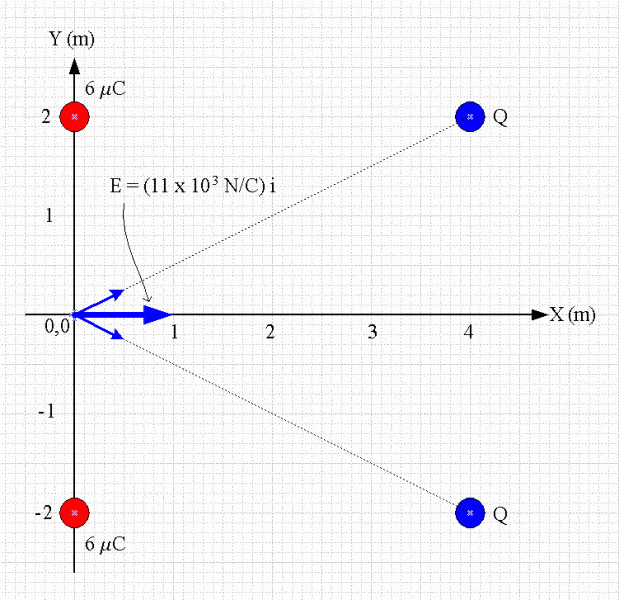# Determine charge Q, given E.

• acdurbin953

## Homework Statement

Charges of 6µC are located at x =0, y = 2.0 m and at x = 0, y = -2.0 m. Charges Q are located at x = 4.0 m, y = 2.0 m and at x = 4.0 m, y = -2.0 m. The electric field at x = 0, y = 0 is (11✕ 103 N/C) i. Determine Q.

E=kq/(r3)

## The Attempt at a Solution

E= kq/(r3)(4m i + 2m j) + kq/(r3)(4m i - 2m j)
E= kq/(r3)(8m i)
E= kq(8m i)/((x2+y2)3/2)
Q= E((x2+y2)3/2)/k(8m)

Plug in all values and Q= 13.6 uC

The correct answer is -13.6 uC... At what point does the negative sign come in, and why?

Does the field have a direction? Do signs of charges have any effect on direction?

The field is in the pos x-direction, and the charges to the left are pos. It seems like if the charges to the left were negative they would impose a greater force in the neg. x-direction, making the net field be in the neg. direction.

Is the answer negative because the negative charges would create a greater attractive force in the pos. direction?

Have a little more faith in yourself and your analysis. Is there anything else going on in the problem statement?

I'm not seeing anything obvious in the problem that would indicate Q being negative, aside from maybe the location of the charges. If instead you say the charges are -4m away from the origin, then the answer comes out correct. My problem is that I don't know why they'd be -4m when they are lying on positive x coordinates.

I'm not seeing anything obvious in the problem that would indicate Q being negative, aside from maybe the location of the charges. If instead you say the charges are -4m away from the origin, then the answer comes out correct. My problem is that I don't know why they'd be -4m when they are lying on positive x coordinates.
The indicator that Q must be negative is that they are located to the right (+x) of the point where they're causing an electric field also pointing to the right.Note that a coordinate system is arbitrary; You could move the origin of the coordinate system without changing the physics in the least. So for example, you might relabel the x-axis so that the 6 μC charges are at x = -23 m, putting the charges Q at -19 m without changing the geometry of the problem.

Usually the simplest way to sort out charge signs and field directions is to make a sketch of the problem and pencil in the field vector due to the charges at the point of interest. Then you can work with the magnitudes of the charges and fields and sort out the signs from the diagram. It can be done symbolically with vector algebra, but that's often more effort than its worth for these sorts of problems.

That graphic made me realize I (foolishly) forgot that the field generated by negative charges points inwards towards the charges. That makes sense then that the charges must be negative. So if Q was a positive charge, then the net E-field at the origin would be in the negative direction, because the charge vectors would point in the opposite direction.